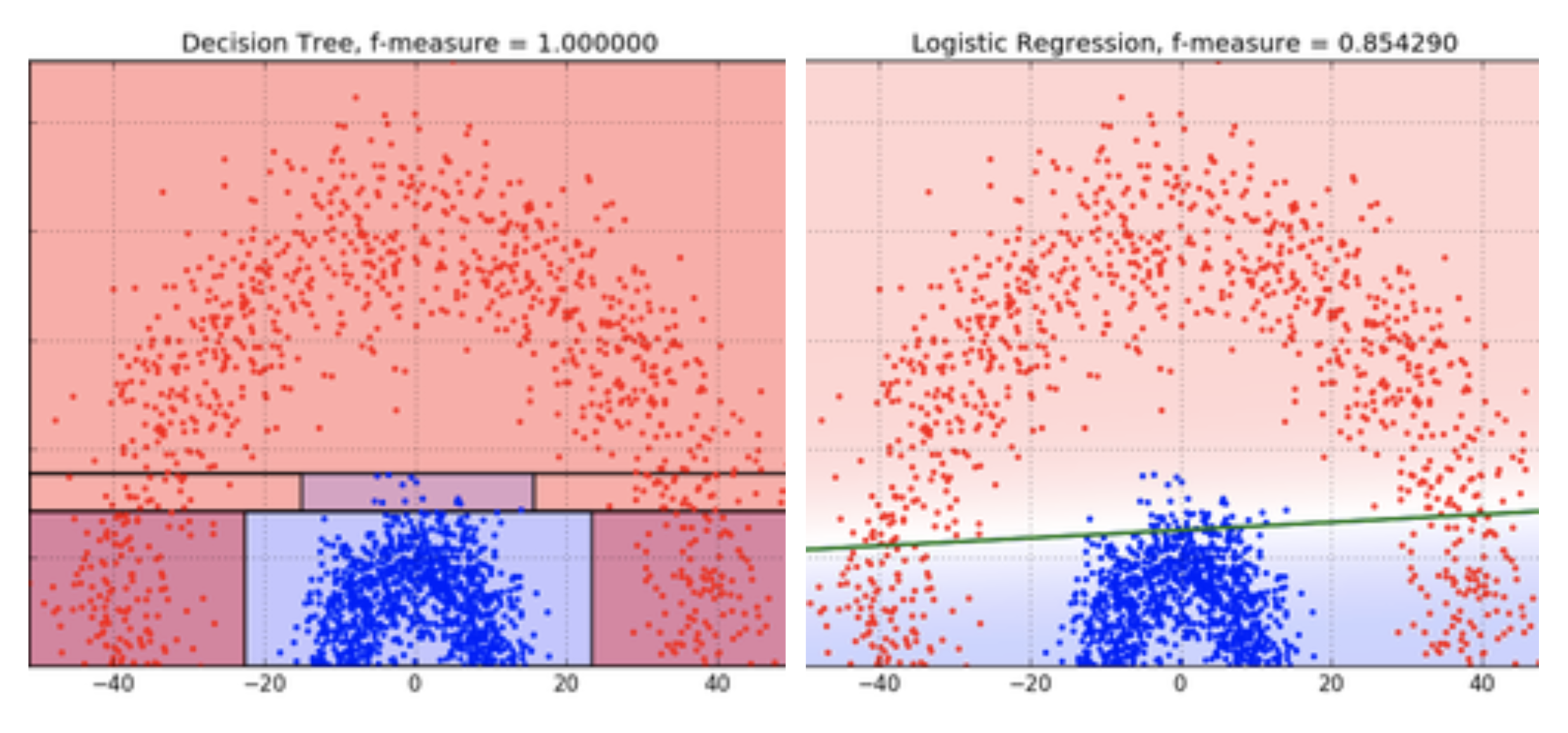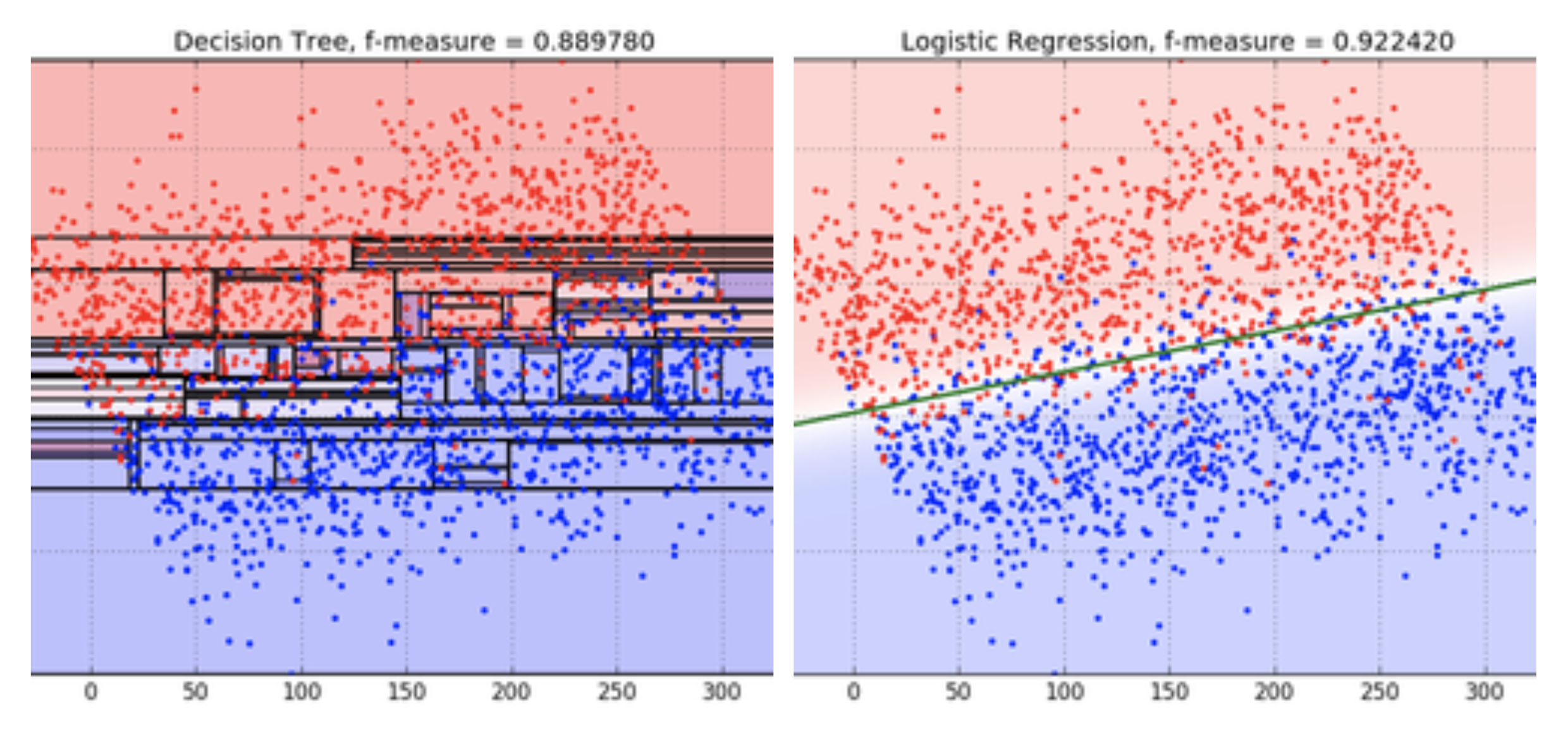## Introduction

Weights & Biases now comes baked into your Kaggle kernels. 🏅

In this report, I'll show you how I used W&B to rank 90 out of 1161 on Kaggle's Categorical Feature Encoding Challenge.

We'll focus on how you can quickly and efficiently narrow down the space of available models and find one that's likely to perform well on a competition using Weights & Biases.

## Not all models are made equal

Unlike Lord of the Rings, in machine learning there is no one ring (model) to rule them all. Different classes of models are good at modeling the underlying patterns of different types of datasets. For instance, decision trees work well in cases where your data has a complex shape:Whereas linear models work best where the dataset is linearly separable:## Model selection in competitive data science vs real world

Before we begin, let’s dive a little deeper into the disparity between model selection in the real world vs for competitive data science.

As William Vorhies said in his blog post “The Kaggle competitions are like formula racing for data science. Winners edge out competitors at the fourth decimal place and like Formula 1 race cars, not many of us would mistake them for daily drivers. The amount of time devoted and the sometimes extreme techniques wouldn’t be appropriate in a data science production environment.”

Kaggle models are indeed like racing cars, they're not built for everyday use. Real world production models are more like a Lexus - reliable but not flashy.

Kaggle competitions and the real world optimize for very different things, with some key differences being:

### Problem Definition

The real world allows you to define your problem and choose the metric that encapsulates the success of your model. This allows you to optimize for a more complex utility function than just a singular metric, where Kaggle competitions come with a single pre-defined metric and don't let you define the problem efficiently.

### Metrics

In the real world we care about inference and training speeds, resource and deployment constraints and other performance metrics, whereas in Kaggle competitions the only thing we care about is the one evaluation metric. Imagine we have a model with 0.98 accuracy that is very resource and time intensive, and another with 0.95 accuracy that is much faster and less compute intensive. In the real world, for a lot of domains we might prefer the 0.95 accuracy model because maybe we care more about the time to inference. In Kaggle competitions, it doesn't matter how long it takes to train the model or how many GPUs it requires, higher accuracy is always better.

### Interpretability

Similarly in the real world, we prefer simpler models that are easier to explain to stakeholders, whereas in Kaggle we pay no heed to model complexity. Model interpretability is important because it allows to take concrete actions to solve the underlying problem. For example, in the real world looking at our model and being able to see a correlation between a feature (e.g. potholes on a street), and the problem (e.g. likelihood of car accident on the street), is more helpful than increasing the prediction accuracy by 0.005%.

### Data Quality

Finally in Kaggle competitions, our dataset is collected and wrangled for us. Anyone who's done data science knows that is almost never the case in real life. But being able to collect and structure our data also gives us more control over the data science process.

### Incentives

All this incentivizes a massive amount of time spent tuning our hyperparameters to extract the last drops of performance from our model, and at times convoluted feature engineer methodologies. While Kaggle competitions are an excellent way to learn data science and feature engineering, they don't address real world concerns like model explainability, problem definition, or deployment constraints.

With that in mind, let's get started with model training. We'll follow the following structure:

• Create a baseline
• Pick a diverse set of initial models, try a few different parameters for each model and choose the strongest contenders
• Hyperparameter Tuning - Use Sweeps to find the best version of the model
• Make the final selection

## Create a Baseline

### Calibration Curve

Calibration Curves plot how well calibrated the predicted probabilities of a classifier are and how to calibrate an uncalibrated classifier. It compares estimated predicted probabilities by a baseline logistic regression model, the model passed as an argument (RandomForest in this case), and by both its isotonic calibration and sigmoid calibrations.

The closer the calibration curves are to a diagonal the better. A transposed sigmoid like curve represents an overfitted classifier, while a sigmoid like curve represents an underfitted classifier. By training isotonic and sigmoid calibrations of the model and comparing their curves we can figure out whether the model is over or underfitting and if so which calibration (sigmoid or isotonic) might help fix this. For more details, check out sklearn's docs.

In this case we can see that vanilla RandomForest suffers from overfitting (as evidenced by the transposed sigmoid curve), potentially because of redundant features (like title) which violate the feature-independence assumption. We can also see that the Logistic Regression model (solid line) was the most effective in fixing this overfitting. We might try training a LogisticRegression model next.

### Class Proportions

Finally we can see from the class proportions plot below that we have an imbalanced dataset with the class 0 in a much larger proportion than class 1.

### ROC Curve

ROC curves plot true positive rate (y-axis) vs false positive rate (x-axis). The ideal score is a TPR = 1 and FPR = 0, which is the point on the top left. Typically we calculate the area under the ROC curve (AUC-ROC), and the greater the AUC-ROC the better.

Here we plot the ROC curves for the values our binary column `target` can take (0 and 1). We can see our model equally good at predicting the probabilities for each of the classes.

### Learning Curve

Learning curves plot cross validated model performance scores vs dataset size, for both training and test sets.

They're useful for catching overfitting/underfitting. Here we can observe that our model is overfitting. While it performs well on the training set right off the bat, the test accuracy never quite achieves parity with the training accuracy.

As we'll see later, this is not the case with some of the other algorithms we tried.

We'll use a RandomForest as our baseline. Next, we'll log the performance of our scikit models using Weights and Biases's built-in integration. You can learn more about the different scikit plots supported by W&B here.

``````def model_algorithm(clf, X_train, y_train, X_test, y_test, name, labels, features):
clf.fit(X_train, y_train)
y_probas = clf.predict_proba(X_test)
y_pred = clf.predict(X_test)
wandb.init(project="kaggle-feature-encoding", name=name, reinit=True)

# Plot learning curve
wandb.sklearn.plot_learning_curve(clf, X_train, y_train)

# Plot confusion matrix
wandb.sklearn.plot_confusion_matrix(y_test, y_pred, labels)

# Plot summary metrics
wandb.sklearn.plot_summary_metrics(clf, X=X_train, y=y_train, X_test=X_test, y_test=y_test)

# Plot class proportions
wandb.sklearn.plot_class_proportions(y_train, y_test, labels)

# Plot calibration curve
wandb.sklearn.plot_calibration_curve(clf, X_train, y_train, name)

# Plot ROC curve
wandb.sklearn.plot_roc(y_test, y_probas, labels)

# Plot precision recall curve
wandb.sklearn.plot_precision_recall(y_test, y_probas, labels)

# Create submission file for kaggle
csv_name = "submission_"+name+".csv"
pd.DataFrame({"id": test_ids, "target": y_pred}).to_csv(csv_name, index=False)
``````

Now we can simply use this function with each model like so:

``````model_algorithm(rf, X_train, y_train, X_test, y_test, 'RandomForest', labels, features)
``````

## Track and compare models

### Pick a diverse set of initial models

Different classes of models are good at modeling different kinds of underlying patterns in data. So a good first step is to quickly test out a few different classes of models to know which ones capture the underlying structure of your dataset most efficiently! Within the realm of our problem type (regression, classification, clustering) we want to try a mixture of tree based, instance based, and kernel based models. Pick a model from each class to test out. We'll talk more about the different model types in the 'models to try' section below.

### Try a few different parameters for each model

While we don't want to spend too much time finding the optimal set of hyper-parameters, we do want to try a few different combinations of hyper-parameters to allow each model class to have the chance to perform well.

### Choose the strongest contenders

We can use the best performing models from this stage to give us intuition around which class of models we want to further dive into. Your Weights and Biases dashboard will guide you to the class of models that performed best for your problem.

## Hyperparameter Tuning - Sweep to find the best version of the model

### Dive deeper into models in the best performing model classes.

Next we select more models belonging to the best performing classes of models we shortlisted above! For example if linear regression seemed to work best, it might be a good idea to try lasso or ridge regression as well.

### Explore the hyper-parameter space in more detail.

At this stage, I'd encourage you to spend some time tuning the hyper-parameters for your candidate models. At the end of this stage you should have the best performing versions of all your strongest models.

## Making the final selection

### Pick final submissions from diverse models.

Ideally we want to select the best models from more than one class of models. This is because if you make your selections from just one class of models and it happens to be the wrong one, all your submissions will perform poorly. Kaggle competitions usually allow you to pick more than one entry for your final submission. I'd recommend choosing predictions made by your strongest models from different classes to build some redundancy into your submissions.

The most important thing to remember is that the public leaderboard is not your friend. Picking you models solely based on your public leaderboard scores will lead to overfitting the training dataset. And when the private leaderboard is revealed after the competition ends, sometimes you might see your rank dropping a lot. You can avoid this little pitfall by using cross-validation when training your models. Then pick the models with the best cross-validation scores, instead of the best leaderboard scores. By doing this you counter overfitting by measuring your model's performance against multiple validation sets instead of just the one subset of test data used by the public leaderboard.

## Final Thoughts - How's this different from making the final selection in the real world?

### Resource constraints

Different models hog different types of resources and knowing whether you’re deploying the models on a IoT/mobile device with a small hard drive and processor or a in cloud can be crucial in picking the right model.

### Training time vs Prediction time vs Accuracy

Knowing what metric(s) you’re optimizing for is also crucial for picking the right model. For instance self driving cars need blazing fast prediction times, whereas fraud detection systems need to quickly update their models to stay up to date with the latest phishing attacks. For other cases like medical diagnosis, we care about the accuracy (or area under the ROC curve) much more than the training times.

More complex models can use orders of magnitude more features to train and make predictions, require more compute but if trained correctly can capture really interesting patterns in the dataset. This also makes them convoluted and harder to explain though. Knowing how important it is to easily to explain the model to stakeholders vs capturing some really interesting trends while giving up explainability is key to picking a model.